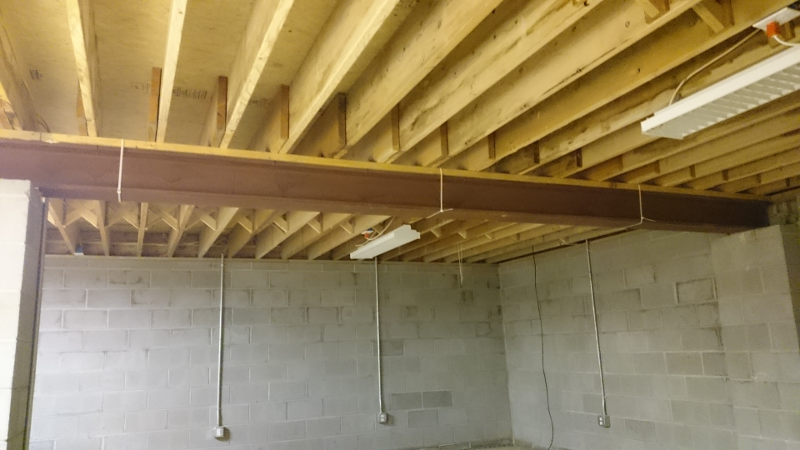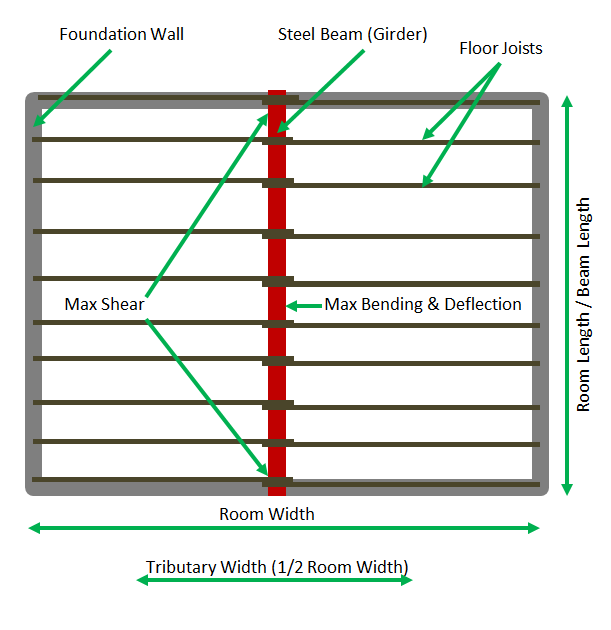# Beam Sizing Problem - Intermediate Complexity Optimization Problem¶

## Story Problem¶

George is building a one-story room addition with a basement onto his house. He is looking to maximize square footage while at the same time meeting his requirements. The addition will have a full basement, and George hates support columns in the middle of his basement. Therefore, George wants to buy a single beam (girder) that will run across the length of his basement down the middle and only be supported on the ends. The beam will be used to support the floor joists. George’s basement will be 8 feet tall, but the beam height will intrude into that space. Therefore, George has decided that he doesn’t want a beam taller than 8 inches. The beam will only support the weights imposed by the single floor, and not the weight of the walls and roof. George has consulted his local building codes and found that a floor must be able to support up to 20psf dead load (furniture, bookshelves, carpet, etc) and up to a 40psf live load (people walking around). George knows that this will be his party room with people jumping around, so George plays it safe and assumes 20psf dead load and 50psf live load (70psf total load). George also consulted his building codes on floor deflection and learned that the floor must not deflect downward more than 1 unit for every 360 unit lengths spanned (a rating of L/360). George knows that this building code minimum will be safe but will result in a very bouncy floor. George hates bouncy floors and has decided to design for a deflection rating of at least L/720. George also wants the length of the room to be greater than or equal to the width of the room.

George knows that the best way to meet his requirements will be to choose a steel wide flange beam. George called his local steel retailer and found that the largest, heaviest, and strongest 8 inch beam they sell is a W8x58 beam, meaning that it is about 8 inches high and weighs 58 pounds per foot length.An actual W8x58 steel beam supporting the floor joists in the basement.

## Objective¶

Maximize room addition square footage. In other words, find the optimum length and width of the room addition while satisfying the constraints. For this exercise, all calculations will be done in inches and pounds.Top view sketch of room addition basement.

## Constraints¶

• Use a W8x58 wide flange beam made from ASTM A992 steel.
• Beam will only be supported at the two ends.
• Achieve a deflection rating of at least L/720.
• Make sure beam safely satisfies bending stress requirements.
• Make sure beam safely satisfies shear stress requirements.
• Room length is greater than room width.

## Constants¶

The constants used in this tutorial are:

from openmdao.api import Problem, ScipyOptimizer, Component, IndepVarComp, Group

E = 29000000 #modulus of elasticity (constant 29000000psi for ASTM A992 Grade 50 steel)
I = 228 #Ix = moment of Inertia (constant 228in4 for the W8x58 beam)
BEAM_WEIGHT_LBS_PER_IN = 58.0 / 12.0 #self weight of beam per unit length (58 lbs/ft or 4.83 lbs/in.)
LIVE_LOAD_PSI = 50.0 / 144 #The live load is 50psf or 0.3472psi.
BEAM_HEIGHT_IN = 8.75 #inches
YIELD_STRENGTH_PSI = 50000 #The maximum yield strength Fy for ASTM A992 Grade 50 steel is 50,000 psi
CROSS_SECTIONAL_AREA_SQIN = 17.1 #sq in


## Room Area Component¶

We want to maximize room area. Room area is given by the following equation.

$\mathrm{room\_area} = \mathrm{room\_length} * \mathrm{room\_width}$

However, in OpenMDAO, problems must be written as a minimization problem. The best way to do that is to negate the equation. Therefore, we want to minimize neg_room_area such that

(1)$\mathrm{neg\_room\_area} = -(\mathrm{room\_length} * \mathrm{room\_width})$

Now we can find our derivatives:

$\frac{d \mathrm{neg\_room\_area}} {d \mathrm{room\_width}} = -\mathrm{room\_length}$$\frac{d \mathrm{neg\_room\_area}} {d \mathrm{room\_length}} = -\mathrm{room\_width}$

Now we can take this equation and create a Component called NegativeArea.

class NegativeArea(Component):

def __init__(self):
super(NegativeArea, self).__init__()

def solve_nonlinear(self, params, unknowns, resids):

room_width = params['room_width']
room_length = params['room_length']

unknowns['neg_room_area'] = -(room_length * room_width)

def linearize(self, params, unknowns, resids):
J = {}

room_width = params['room_width']
room_length = params['room_length']

J['neg_room_area','room_width'] = -room_length
J['neg_room_area','room_length'] = -room_width

return J


## Room Length and Width Component¶

George wants the length of the room to be at least the width of the room, given by the following equation.

$\mathrm{room\_length} \geq \mathrm{room\_width}$

If we create a variable called length_minus_width, we can constrain it to be greater than or equal to zero.

(2)$\mathrm{length\_minus\_width} = \mathrm{room\_length} - \mathrm{room\_width} \geq 0$

Now we can find our derivatives:

$\frac{d \mathrm{length\_minus\_width}} {d \mathrm{room\_width}} = -1$$\frac{d \mathrm{length\_minus\_width}} {d \mathrm{room\_length}} = 1$

Now we can take this equation and create a Component called LengthMinusWidth.

class LengthMinusWidth(Component):

def __init__(self):
super(LengthMinusWidth, self).__init__()

def solve_nonlinear(self, params, unknowns, resids):

room_width = params['room_width']
room_length = params['room_length']

unknowns['length_minus_width'] = room_length - room_width

def linearize(self, params, unknowns, resids):
J = {}

room_width = params['room_width']
room_length = params['room_length']

J['length_minus_width','room_width'] = -1.0
J['length_minus_width','room_length'] = 1.0

return J


## Deflection Component¶

Maximum deflection for a uniformly loaded beam can be calculated as

$\delta = \frac{5 q L^4}{(E I_x 384)}$

where:

• $$\delta$$ = maximum deflection (in)
• E = modulus of elasticity (constant 29000000psi for ASTM A992 Grade 50 steel)
• q = uniform load per unit length (lb/in)
• L = length of beam = room_length
• $$I_x$$ = moment of Inertia (constant 228in4 for the W8x58 beam)

q can be calculated by:

$q = (\mathrm{tributary\_width})*(\mathrm{dead\_load} + \mathrm{live\_load}) + \mathrm{self\_weight\_of\_beam\_per\_unit\_length}$

$q = (0.5 * \mathrm{TOTAL\_LOAD\_PSI} * \mathrm{room\_width}) + \mathrm{BEAM\_WEIGHT\_LBS\_PER\_IN}$

Since George wants a deflection rating of at least L/720, our first constraint can be written as:

$\mathrm{deflection} = \frac{L}{\delta} \geq 720$
$\mathrm{deflection} = \frac{E * I_x * 384}{5 * q * L^3} \geq 720$

Substituting for q, and since the length of the beam is the room_length in our case:

(3)$\mathrm{deflection} = \frac{E * I_x * 384}{5 * ((0.5 * \mathrm{TOTAL\_LOAD\_PSI} * \mathrm{room\_width}) + \mathrm{BEAM\_WEIGHT\_LBS\_PER\_IN}) * \mathrm{room\_length}^3} \geq 720$

Now we can find our derivatives:

$\frac{d \mathrm{deflection}} {d \mathrm{room\_width}} = \frac{-192 * E * I * \mathrm{TOTAL\_LOAD\_PSI}} {5 * \mathrm{room\_length}^3 * (\mathrm{TOTAL\_LOAD\_PSI} * \frac{\mathrm{room\_width}}{2} + \mathrm{BEAM\_WEIGHT\_LBS\_PER\_IN)}^2}$$\frac{d \mathrm{deflection}} {d \mathrm{room\_length}} = \frac{-1152 * E * I} {5 * (\frac{\mathrm{TOTAL\_LOAD\_PSI} * \mathrm{room\_width} }{2} + \mathrm{BEAM\_WEIGHT\_LBS\_PER\_IN}) * \mathrm{room\_length}^4 }$

Now we can take this equation and create a Component called Deflection.

class Deflection(Component):

def __init__(self):
super(Deflection, self).__init__()

def solve_nonlinear(self, params, unknowns, resids):

room_width = params['room_width']
room_length = params['room_length']

unknowns['deflection'] = (E * I * 384.0) / (5.0 * ((0.5 * TOTAL_LOAD_PSI * room_width)  + BEAM_WEIGHT_LBS_PER_IN) * room_length**3)

def linearize(self, params, unknowns, resids):
J = {}

room_width = params['room_width']
room_length = params['room_length']

J['deflection','room_width'] = (-192.0 * E * I * TOTAL_LOAD_PSI) / ((5.0 * room_length**3) * (TOTAL_LOAD_PSI * room_width/2.0 + BEAM_WEIGHT_LBS_PER_IN)**2)
J['deflection','room_length'] = (-1152.0 * E * I) / (5.0 * ((TOTAL_LOAD_PSI * room_width)/2.0 + BEAM_WEIGHT_LBS_PER_IN) * room_length**4)

return J


## Bending Stress Component¶

Deflection is usually the limiting factor in beam design since designing just to the maximum load would result in an unacceptable deflection. However, it is important to be safe by calculating the maximum bending stress of the beam. Maximum stress in a beam with uniform load supported at both ends can be calculated as

$\sigma = \frac{y q L^2} {8 I_x}$

where:

• $$\sigma$$ = maximum stress (psi)
• y = Distance of extreme point off neutral axis (0.5*beam_height)

The maximum yield strength Fy for ASTM A992 Grade 50 steel is 50,000 psi. George wants a safety factor of 2.0 in his design, so:

$\begin{split}\mathrm{bending\_stress\_ratio} = \frac{\sigma} {\mathrm{YIELD\_STRENGTH\_PSI}} < 0.5\end{split}$

Substituting for $$\sigma$$, we get

$\begin{split}\mathrm{bending\_stress\_ratio} = \frac{y * q * L^2} {8 * \mathrm{YIELD\_STRENGTH\_PSI} * I_x} < 0.5\end{split}$
(4)$\mathrm{bending\_stress\_ratio} = \frac{0.5 * \mathrm{BEAM\_HEIGHT\_IN} * ((0.5 * \mathrm{TOTAL\_LOAD\_PSI} * \mathrm{room\_width}) + \mathrm{BEAM\_WEIGHT\_LBS\_PER\_IN}) * \mathrm{room\_length}^2} {8 * \mathrm{YIELD\_STRENGTH\_PSI} * I_x}$

Now we can find our derivatives:

$\frac{d \mathrm{bending\_stress\_ratio}} {d \mathrm{room\_width}} = \frac{\mathrm{room\_length}^2 * \mathrm{BEAM\_HEIGHT\_IN} * (\mathrm{TOTAL\_LOAD\_PSI}*\mathrm{room\_width}/2 + \mathrm{BEAM\_WEIGHT\_LBS\_PER\_IN})} {16I_x * \mathrm{YIELD\_STRENGTH\_PSI}}$$\frac{d \mathrm{bending\_stress\_ratio}} {d \mathrm{room\_length}} = \frac{(\mathrm{BEAM\_WEIGHT\_LBS\_PER\_IN} + (\mathrm{TOTAL\_LOAD\_PSI}*\mathrm{room\_width}/2)) * \mathrm{BEAM\_HEIGHT\_IN} * \mathrm{room\_length}} {8I_x * \mathrm{YIELD\_STRENGTH\_PSI}}$

Now we can take this equation and create a Component called BendingStress.

class BendingStress(Component):

def __init__(self):
super(BendingStress, self).__init__()

def solve_nonlinear(self, params, unknowns, resids):

room_width = params['room_width']
room_length = params['room_length']

unknowns['bending_stress_ratio'] = (0.5*BEAM_HEIGHT_IN * ((0.5 * TOTAL_LOAD_PSI * room_width)  + BEAM_WEIGHT_LBS_PER_IN) * (room_length)**2) / (8.0 * YIELD_STRENGTH_PSI * I)

def linearize(self, params, unknowns, resids):
J = {}

room_width = params['room_width']
room_length = params['room_length']

J['bending_stress_ratio','room_width'] = (room_length**2) * BEAM_HEIGHT_IN * (TOTAL_LOAD_PSI*room_width/2.0 + BEAM_WEIGHT_LBS_PER_IN) / (16.0 * I * YIELD_STRENGTH_PSI)
J['bending_stress_ratio','room_length'] = (BEAM_WEIGHT_LBS_PER_IN + (TOTAL_LOAD_PSI*room_width/2.0)) * BEAM_HEIGHT_IN * room_length / (8.0 * I * YIELD_STRENGTH_PSI)

return J


## Shear Stress Component¶

In addition to making sure the bending stress is safe, it is also important to make sure the shear stress is safe. According to http://www.wikiengineer.com/Structural/SteelBeamShearStrength:

It is important to know that shear force will normally not govern over bending force, unless the member in question is very short in length, with very high loads. This is due to the fact that the bending stress will normally increase exponentially with the length of a beam while shear stress will only increase if the Force acting on the beam is increased.”

The max sheer force V in pounds for a uniformly distributed beam supported at the ends is

$V = 0.5*\mathrm{total\_weight} = 0.5qL.$

The max shear stress fv on the beam in psi is

$f_v = \frac{V}{A}$

where A is the cross sectional area of the beam.

The max shear stress $$f_v$$ should never exceed our maximum yield strength $$F_y = 50,000psi$$. However, a safety factor of 3 is recommended for sheer stress design. Therefore, we can write:

$\begin{split}\mathrm{shear\_stress\_ratio} = \frac{f_v}{F_y} < \frac{1}{3}\end{split}$$\begin{split}\mathrm{shear\_stress\_ratio} = \frac{0.5qL}{A F_y} < \frac{1}{3}\end{split}$
(5)$\mathrm{shear\_stress\_ratio} = \frac{0.5 * ((0.5 * \mathrm{TOTAL\_LOAD\_PSI} * \mathrm{room\_width}) + \mathrm{BEAM\_WEIGHT\_LBS\_PER\_IN}) * \mathrm{room\_length} }{\mathrm{CROSS\_SECTIONAL\_AREA\_SQIN} * \mathrm{YIELD\_STRENGTH\_PSI}}$

Now we can find our derivatives:

$\frac{d \mathrm{shear\_stress\_ratio}} {d \mathrm{room\_width}} = \frac{\mathrm{TOTAL\_LOAD\_PSI} * \mathrm{room\_length}} {4 * \mathrm{YIELD\_STRENGTH\_PSI} * \mathrm{CROSS\_SECTIONAL\_AREA\_SQIN}}$$\frac{d \mathrm{shear\_stress\_ratio}} {d \mathrm{room\_length}} = \frac{\mathrm{BEAM\_WEIGHT\_LBS\_PER\_IN} + (\mathrm{TOTAL\_LOAD\_PSI} * \mathrm{room\_width} / 2)} {2 * \mathrm{YIELD\_STRENGTH\_PSI} * \mathrm{CROSS\_SECTIONAL\_AREA\_SQIN}}$

Now we can take this equation and create a Component called ShearStress.

class ShearStress(Component):

def __init__(self):
super(ShearStress, self).__init__()

def solve_nonlinear(self, params, unknowns, resids):

room_width = params['room_width']
room_length = params['room_length']

unknowns['shear_stress_ratio'] = 0.5 * ((0.5 * TOTAL_LOAD_PSI * room_width)  + BEAM_WEIGHT_LBS_PER_IN) * (room_length) / (CROSS_SECTIONAL_AREA_SQIN * YIELD_STRENGTH_PSI)

def linearize(self, params, unknowns, resids):
J = {}

room_width = params['room_width']
room_length = params['room_length']

J['shear_stress_ratio','room_width'] = TOTAL_LOAD_PSI * room_length / (4.0 * YIELD_STRENGTH_PSI * CROSS_SECTIONAL_AREA_SQIN)
J['shear_stress_ratio','room_length'] = (BEAM_WEIGHT_LBS_PER_IN + (TOTAL_LOAD_PSI * room_width / 2.0))/(2.0 * YIELD_STRENGTH_PSI * CROSS_SECTIONAL_AREA_SQIN)

return J


## Putting it all Together¶

First we must take all five of our Components and combine them into a Group. The design variables room_length and room_width must be created as IndepVarComp, and they are initialized to 100 inches as a best guess. Then, we connnect the design variables to the inputs of the five Components.

class BeamTutorial(Group):

def __init__(self):
super(BeamTutorial, self).__init__()

#make connections from design variables to the Components
self.connect('ivc_rlength.room_length','d_len_minus_wid.room_length')
self.connect('ivc_rwidth.room_width','d_len_minus_wid.room_width')

self.connect('ivc_rlength.room_length','d_deflection.room_length')
self.connect('ivc_rwidth.room_width','d_deflection.room_width')

self.connect('ivc_rlength.room_length','d_bending.room_length')
self.connect('ivc_rwidth.room_width','d_bending.room_width')

self.connect('ivc_rlength.room_length','d_shear.room_length')
self.connect('ivc_rwidth.room_width','d_shear.room_width')

self.connect('ivc_rlength.room_length','d_neg_area.room_length')
self.connect('ivc_rwidth.room_width','d_neg_area.room_width')


Finally, we set up the problem. We bound room_length to only be between 5ft and 50ft, and room_width to be between 5ft and 30ft. We set our minimization objective to neg_room_area. Then we constrain the outputs from our Components.

top = Problem()
top.root = BeamTutorial()

top.driver = ScipyOptimizer()
top.driver.options['optimizer'] = 'SLSQP'
top.driver.options['tol'] = 1.0e-8
top.driver.options['maxiter'] = 10000 #maximum number of solver iterations

#room length and width bounds
top.driver.add_desvar('ivc_rlength.room_length', lower=5.0*12.0, upper=50.0*12.0) #domain: 1in <= length <= 50ft
top.driver.add_desvar('ivc_rwidth.room_width', lower=5.0*12.0, upper=30.0*12.0) #domain: 1in <= width <= 30ft

top.driver.add_objective('d_neg_area.neg_room_area') #minimize negative area (or maximize area)

top.setup()
top.run()

print("\n")
print( "Solution found")
print("room area: %f in^2 (%f ft^2)" % (-top['d_neg_area.neg_room_area'], -top['d_neg_area.neg_room_area']/144.0))
print("room width: %f in (%f ft)" % (top['ivc_rwidth.room_width'], top['ivc_rwidth.room_width']/12.0))
print("room/beam length: %f in (%f ft)" % (top['ivc_rlength.room_length'], top['ivc_rlength.room_length']/12.0))
print( "deflection: L/%f"  % (top['d_deflection.deflection']))
print( "bending stress ratio: %f"  % (top['d_bending.bending_stress_ratio']))
print( "shear stress ratio: %f"  % (top['d_shear.shear_stress_ratio']))

print( "Finished!")


## Output¶

Solution found

room area: 51655.257618 in^2 (358.717067 ft^2)

room width: 227.277956 in (18.939830 ft)

room/beam length: 227.277904 in (18.939825 ft)

deflection: L/719.999555

bending stress ratio: 0.148863

shear stress ratio: 0.007985